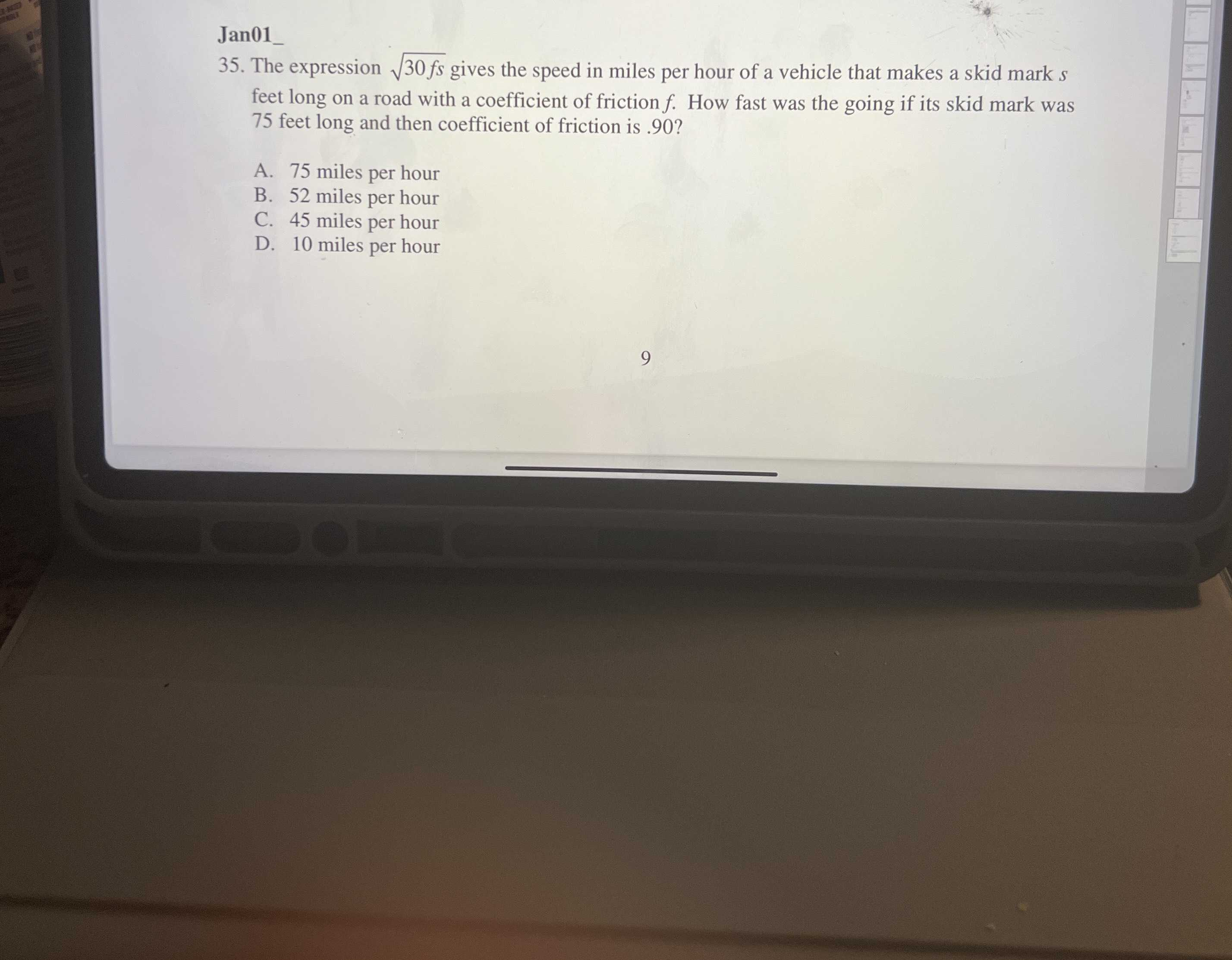### Still have math questions?

Arithmetic
Question35. The expression $$\sqrt { 30 f s }$$ gives the speed in miles per hour of a vehicle that makes a skid mark $$s$$ feet long on a road with a coefficient of friction $$f$$ . How fast was the going if its skid mark was

$$75$$ feet long and then coefficient of friction is $$.90$$ ? A. $$75$$ miles per hour B. $$52$$ miles per hour C. $$45$$ miles per hour D. $$10$$ miles per hour